# Double mapping cylinder

(diff) ← Older revision | Latest revision (diff) | Newer revision → (diff)
Suppose$X,Y,Z$ are topological spaces and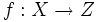$f:X \to Z$ and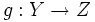$g: Y \to Z$ are continuous maps. The double mapping cylinder of$f$ and$g$ is defined as the quotient of$X \times [0,1] \sqcup Y \sqcup Z$ via the relations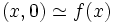$(x,0) \simeq f(x)$ and$(x,1) \simeq g(x)$.
• Mapping cylinder: Here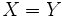$X = Y$ and$f$ is the identity map
• Mapping cone: Here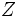$Z$ is a one-point space and$f$ is the map to that one point
• Join: The join of spaces$A$ and$B$ is the double mapping cylinder where$X = A \times B$,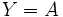$Y = A$,$Z = B$ and the maps are simply projections onto the coordinates